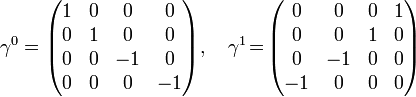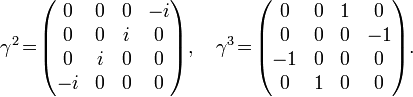## What the "i" represents, is Natural?

A Conformal Diagram of a Minkowski Spacetime
John D. Norton

First, here is the conformal diagram of a Minkowski spacetime. This is the complete spacetime. It includes all of the infinity of space and the infinity of time through which things persist.

This diagram gives the simplest case in which we consider just one dimension of space.

Note the three types of infinities: timelike, lightlike and spacelike. They correspond to the different vanishing points in an ordinary perspective drawing.

***
The Quantum Theory of the ElectronPaul Dirac

Projective Geometry

When one is doing mathematical work, there are essentially two different ways of thinking about the subject: the algebraic way, and the geometric way. With the algebraic way, one is all the time writing down equations and following rules of deduction, and interpreting these equations to get more equations. With the geometric way, one is thinking in terms of pictures; pictures which one imagines in space in some way, and one just tries to get a feeling for the relationships between the quantities occurring in those pictures. Now, a good mathematician has to be a master of both ways of those ways of thinking, but even so, he will have a preference for one or the other; I don’t think he can avoid it. In my own case, my own preference is especially for the geometrical way.

***

In mathematical physics, the gamma matrices, {γ0123}, also known as the Dirac matrices, are a set of conventional matrices with specific anticommutation relations that ensure they generate a matrix representation of the Clifford algebra Cℓ(1,3). It is also possible to define higher-dimensional Gamma matrices. When interpreted as the matrices of the action of a set of orthogonal basis vectors for contravariant vectors in Minkowski space, the column vectors on which the matrices act become a space of spinors, on which the Clifford algebra of space time acts. This in turn makes it possible to represent infinitesimal spatial rotations and Lorentz boosts. Spinors facilitate space-time computations in general, and in particular are fundamental to the Dirac equation for relativistic spin-½ particles.

In Dirac representation, the four contravariant gamma matrices are$\gamma^0 = \begin{pmatrix} 1 & 0 & 0 & 0 \\ 0 & 1 & 0 & 0 \\ 0 & 0 & -1 & 0 \\ 0 & 0 & 0 & -1 \end{pmatrix},\quad \gamma^1 \!=\! \begin{pmatrix} 0 & 0 & 0 & 1 \\ 0 & 0 & 1 & 0 \\ 0 & -1 & 0 & 0 \\ -1 & 0 & 0 & 0 \end{pmatrix}$$\gamma^2 \!=\! \begin{pmatrix} 0 & 0 & 0 & -i \\ 0 & 0 & i & 0 \\ 0 & i & 0 & 0 \\ -i & 0 & 0 & 0 \end{pmatrix},\quad \gamma^3 \!=\! \begin{pmatrix} 0 & 0 & 1 & 0 \\ 0 & 0 & 0 & -1 \\ -1 & 0 & 0 & 0 \\ 0 & 1 & 0 & 0 \end{pmatrix}.$

Analogue sets of gamma matrices can be defined in any dimension and signature of the metric. For example the Pauli matrices are a set of “gamma” matrices in dimension 3 with metric of Euclidean signature (3,0).

***

### Thoughts on Angel and Demons PlotFor me, the most attractive way … would be to capture the antihydrogen in a neutral particle trap … The objective would be to then study the properties of a small number of [antihydrogen] atoms confined in the neutral trap for a long time.“Gerald Gabrielse, 1986 Erice Lecture (shortly after first trapping of antiprotons)
“Penning Traps, Masses and Antiprotons”, in Fundamental Symmetries,
edited by P. Bloch, P. Paulopoulos and R. Klapisch, p. 59 (Plenum, New York, 1987). See:Goals for ATRAP

***

Physics at this high energy scale describes the universe as it existed during the first moments of the Big Bang. These high energy scales are completely beyond the range which can be created in the particle accelerators we currently have (or will have in the foreseeable future.) Most of the physical theories that we use to understand the universe that we live in also break down at the Planck scale. However, string theory shows unique promise in being able to describe the physics of the Planck scale and the Big Bang.

This entry was posted in Dirac and tagged . Bookmark the permalink.